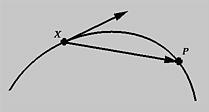# Tangent Vector and Tangent LineConsider a fixed point X and a moving point P on a curve. As point P moves toward X, the vector from X to P approaches the tangent vector at X. The line that contains the tangent vector is the tangent line.Computing the tangent vector at a point is very simple. Recall from your calculus knowledge that the derivative of parametric curve f(u) is the following:

f'(u) = ( f'(u), g'(u), h'(u) )
where f'(u) = df/du, g'(u) = dg/du and h'(u) = dh/du.

In general, the length of the tangent vector f'(u) is not one, and normalization is required. That is, the unit-length tangent vector at parameter u, or at point f(u), is

f'(u) / | f'(u) |
where | x | is the length of vector x. The tangent line at f(u) is either
f(u) + tf'(u)
or if unit-length direction vector is preferred
f(u) + t(f'(u)/|f'(u)|)
where t is a parameter.### Examples

• Consider the circle f(u) = ( rcos(2*PI*u) + p, rsin(2*PI*u) + q ), where u is in the range of 0 and 1. We have the tangent vector at u as follows:
f'(u) = ( -2*PI*rsin(2*PI*u), 2*PI*rcos(2*PI*u) )
and the tangent line at f(u):
f(u) + tf'(u) = ( rcos(2*PI*u) + p, rsin(2*PI*u) + q ) + t ( -2*PI*rsin(2*PI*u), 2*PI*rcos(2*PI*u)
• Consider a space cubic curve f(u) = ( u, u2, u3 ). We have tangent vector f'(u) = ( 1, 2u, 3u2 ) and tangent line f(u) + tf'(u) = ( u + t, u2 + 2tu, u3 + 3tu2 ), where t is the line parameter.
• The circular helix curve has an equation as follows:
f(u) = ( acos(u), asin(u), bu )
It has tangent vector
f'(u) = ( -asin(u), acos(u), b )
and tangent line
f(u) + tf'(u) = ( a(cos(u) - tsin(u)), a(sin(u) + tcos(u)), b(t+u) )